Homework Help Question & Answers

# Consider the molecule CF, in which the vibrational energy is 1285.77 cm-1. The temperature is 630.0...

Consider the molecule CF, in which the vibrational energy is 1285.77 cm-1. The temperature is 630.0 K. Assume that the molecule has constant vibrational energy spacing as described in the practice version of this question. Calculate the ratio of the population in the first excited state (n=1) to that in the ground state (n=0).

N1/N0=

Calculate the ratio of the population in the second excited state (n=2) to that in the ground state.

N2/N0=

Now calculate the ratio of the population in the first excited state to that in the ground state if the temperature increases to 880.0 K.

N1/N0=

Please Show All Work - for Phsyical Chemistry 2, Thank you!

#### Homework Answers

Answer #1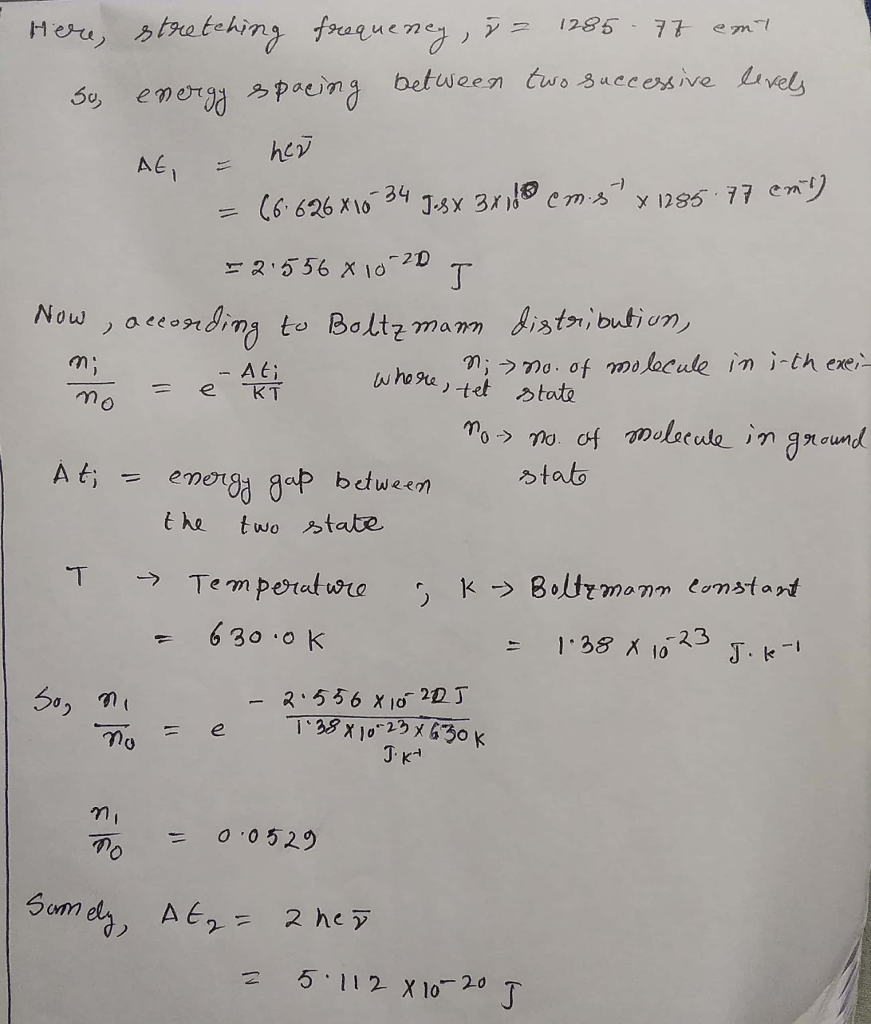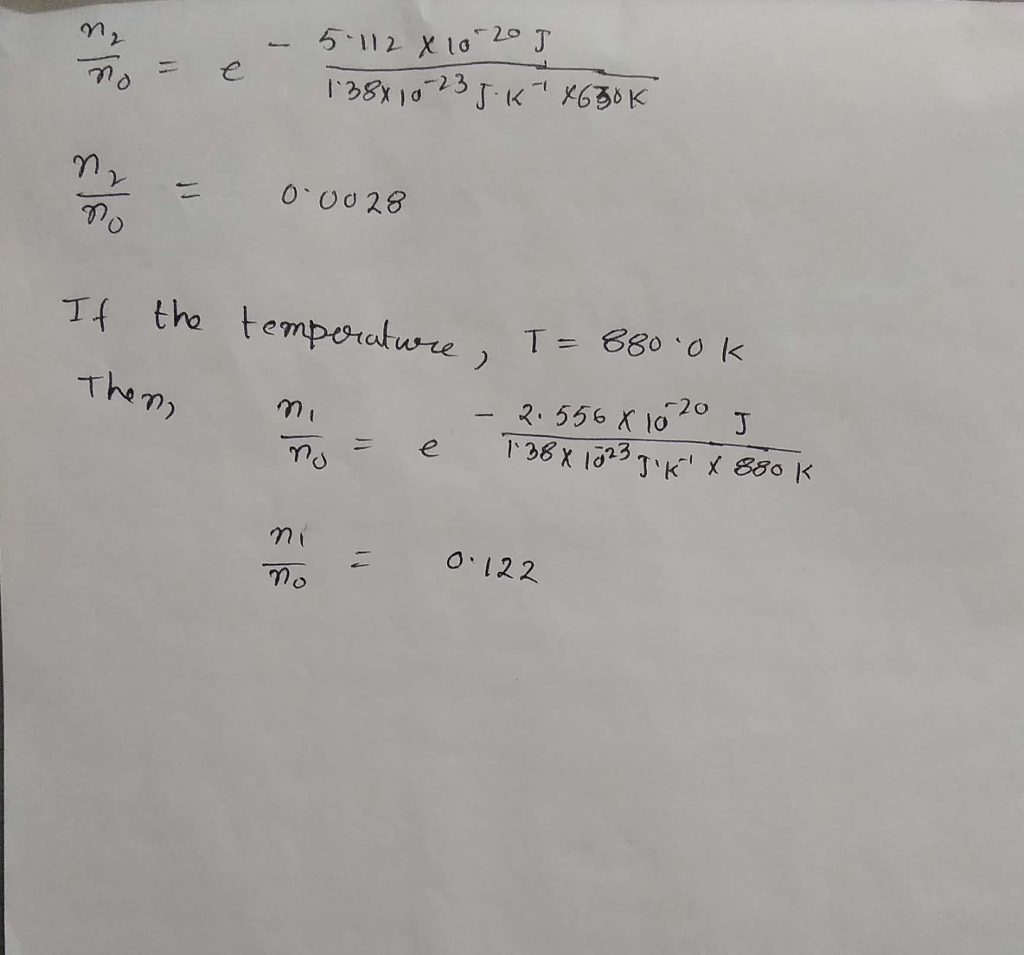If you still have any query, feel free to ask in the comments. If you like my work, please give a thumbs up.

Know the answer?
Your Answer:

#### Post as a guest

Your Name:

What's your source?

#### Earn Coin

Coins can be redeemed for fabulous gifts.

Not the answer you're looking for? Ask your own homework help question. Our experts will answer your question WITHIN MINUTES for Free.
Similar Homework Help Questions
• ### Calculate the ratio of HBr molecules in the first excited vibrational state compared to the ground state at 800K. Remember that finding the probability of finding a molecule in a given state, i, is re...

Calculate the ratio of HBr molecules in the first excited vibrational state compared to the ground state at 800K. Remember that finding the probability of finding a molecule in a given state, i, is related to its energy, Ei, by the following equation: P(n) e^(-En/KT) You will need the following information to calculate the energy of the vibrational states: En = hv(n+1/2) for HBr v= 7.944*10^14s^-1 K=1.3807*10^-24J/K h=6.626*10^-34J*s

• ### 3. Consider a canonical system with uniformly spaced energy levels (spacing = €). The populations of...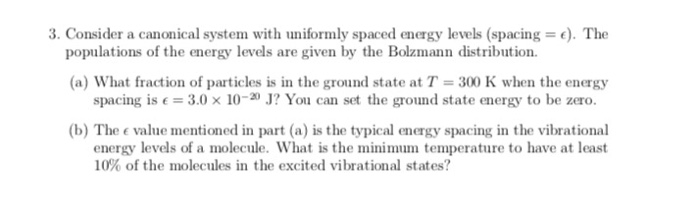3. Consider a canonical system with uniformly spaced energy levels (spacing = €). The populations of the energy levels are given by the Bolzmann distribution. (a) What fraction of particles is in the ground state at T = 300 K when the energy spacing is € = 3.0 x 10-20 J? You can set the ground state energy to be zero. (b) The e value mentioned in part (a) is the typical energy spacing in the vibrational energy levels of...

• ### 3. Consider a canonical system with uniformly spaced energy levels (spacing = e). The populations of...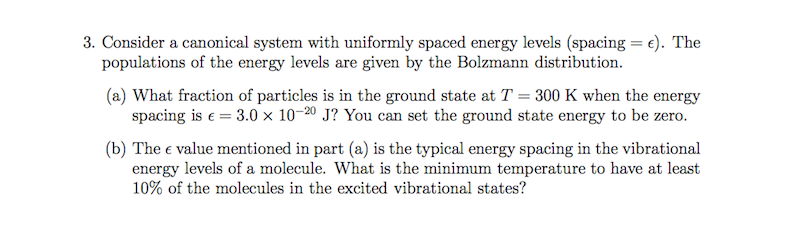3. Consider a canonical system with uniformly spaced energy levels (spacing = e). The populations of the energy levels are given by the Bolzmann distribution (a) What fraction of particles is in the ground state at T 300 K when the energy spacing is e 3.0 x 10-20 J? You can set the ground state energy to be zero. (b) The e value mentioned in part (a) is the typical energy spacing in the vibrational energy levels of a molecule....

• ### A) Which vibrational mode is degenerate? B) Draw all vibrational modes. C) Calculate the zero point...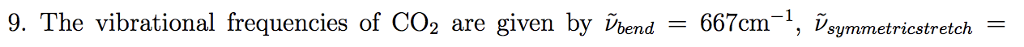A) Which vibrational mode is degenerate? B) Draw all vibrational modes. C) Calculate the zero point vibration energy of CO2 D) Calculate the energy of the lowest vibrationally excited state E) Calculate the ratio of the molecules in the lowest vibrationally excited state and the vibrational ground state at temperature of 300K 9. The vibrational frequencies of CO2 are given by Pond-667cm-l, Vsymmetricstretch

• ### 18-22. In analogy to the characteristic vibrational temperature, we can define a characteristic electronic temperature by...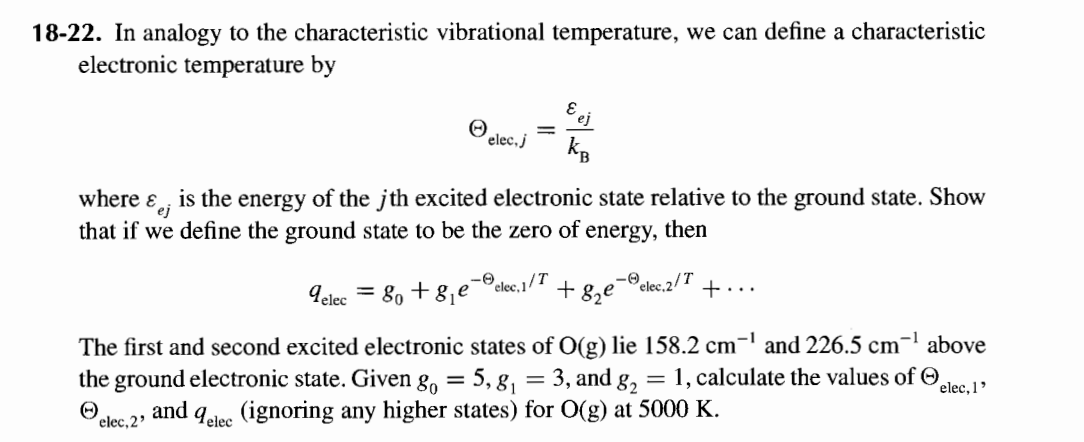18-22. In analogy to the characteristic vibrational temperature, we can define a characteristic electronic temperature by @ele, s = where is the energy of the jth excited electronic state relative to the ground state. Show that if we define the ground state to be the zero of energy, then Telee = 8+8, e-Ociec.1/7 +8,e-Pelec.2/T +... The first and second excited electronic states of O(g) lie 158.2 cm-' and 226.5 cm- above the ground electronic state. Given 8, = 5,8, =...

• ### i need a solution for these provlems. plz~~ 3) The vibrational energy of a carbon monoxide,...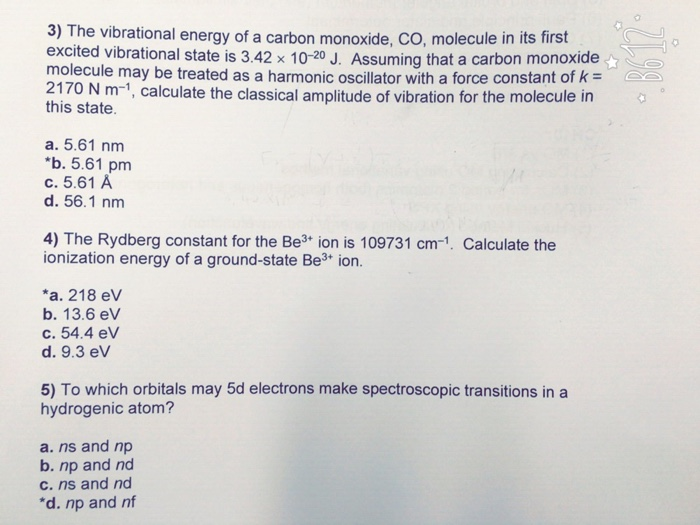i need a solution for these provlems. plz~~ 3) The vibrational energy of a carbon monoxide, co, molecule in its first excited vibrational state is 3.42 x 10-20 J. Assuming that a carbon monoxide molecule may be treated as a harmonic oscillator with a force constant of k 2170 N m-1, calculate the classical amplitude of vibration for the molecule in this state. a. 5.61 nm *b. 5.61 pm c. 5.61 A d. 56.1 nm 4) The Rydberg constant for...

• ### Vibrational spectrum of H-CI: theory Calculate the vibrational amplitude of a state: al amplitude of a...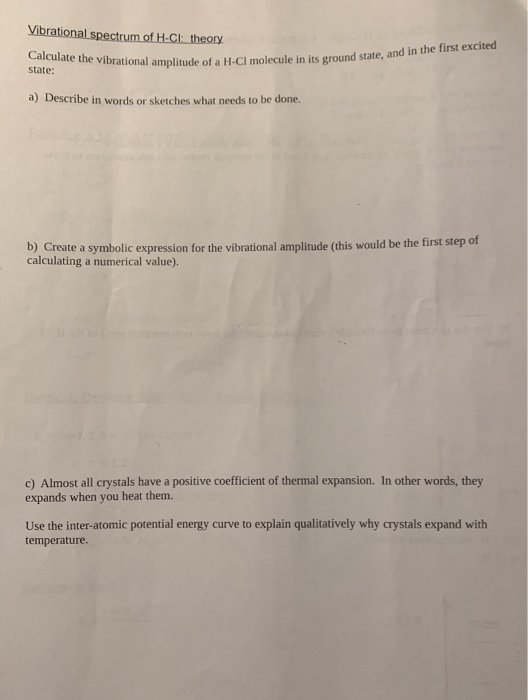Vibrational spectrum of H-CI: theory Calculate the vibrational amplitude of a state: al amplitude of a H-Cl molecule in its ground state, and in the first excited a) Describe in words or sketches what needs to be done. b) Create a symbolic expression for the vibrational amplitude (this would be the first step of calculating a numerical value). c) Almost all crystals have a positive coefficient of thermal expansion. In other words, they expands when you heat them. Use the...

• ### Quantum, 1D harmonic oscillator. Please answer in full. Thanks. Q3. The energy levels of the 1D harmonic oscillator are given by: En = (n +2)ha, n=0. 1, 2, 3, The CO molecule has a (reduced) mass of...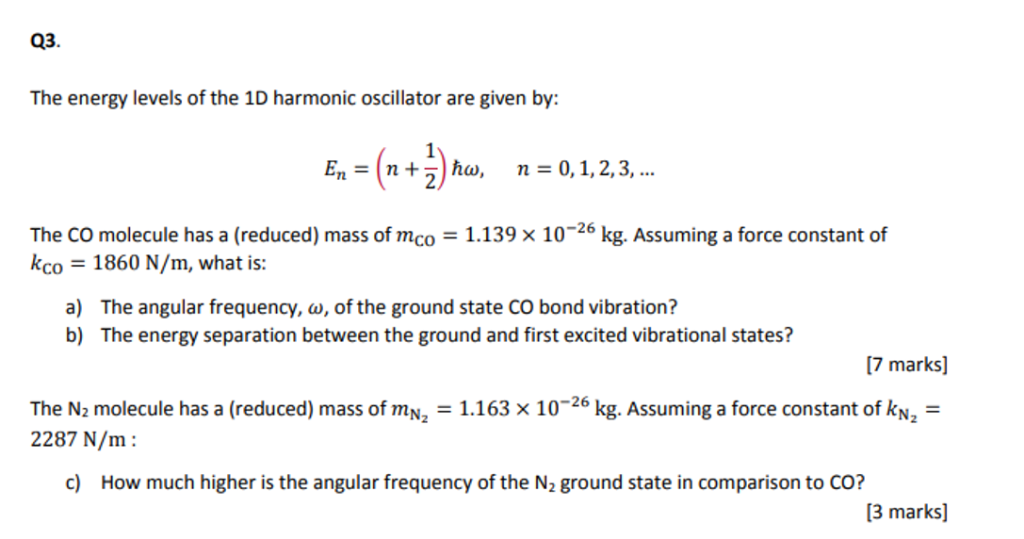Quantum, 1D harmonic oscillator. Please answer in full. Thanks. Q3. The energy levels of the 1D harmonic oscillator are given by: En = (n +2)ha, n=0. 1, 2, 3, The CO molecule has a (reduced) mass of mco = 1.139 × 10-26 kg. Assuming a force constant of kco 1860 N/m, what is: a) The angular frequency, w, of the ground state CO bond vibration? b) The energy separation between the ground and first excited vibrational states? 7 marks] The...

• ### Consider a gas of diatomic molecules (moment of inertia I) at an absolute temperature T. If Eg is a ground-state energy...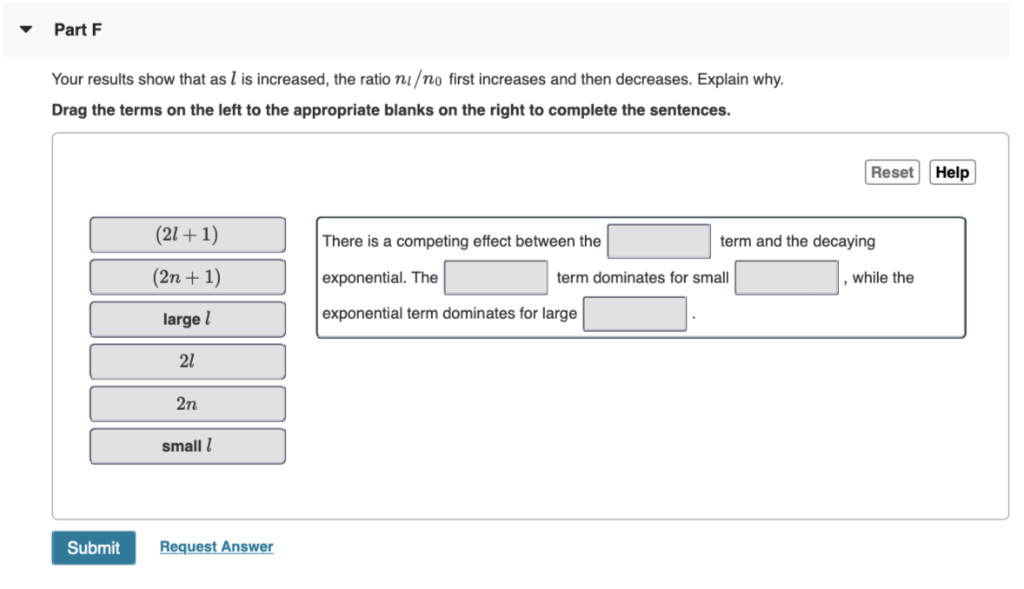Consider a gas of diatomic molecules (moment of inertia I) at an absolute temperature T. If Eg is a ground-state energy and Eex is the energy of an excited state, then the Maxwell-Boltzmann distribution predicts that the ratio of the numbers of molecules in the two states is nexng=e−(Eex−Eg)/kT. The ratio of the number of molecules in the lth rotational energy level to the number of molecules in the ground-state (l=0) rotational level is nln0=(2l+1)e−l(l+1)ℏ2/2IkT. The moment of inertia of...

• ### 4&5 only thnkyouu :) 3. The force constant for 119F molecule is 966 N/m. a) Calculate...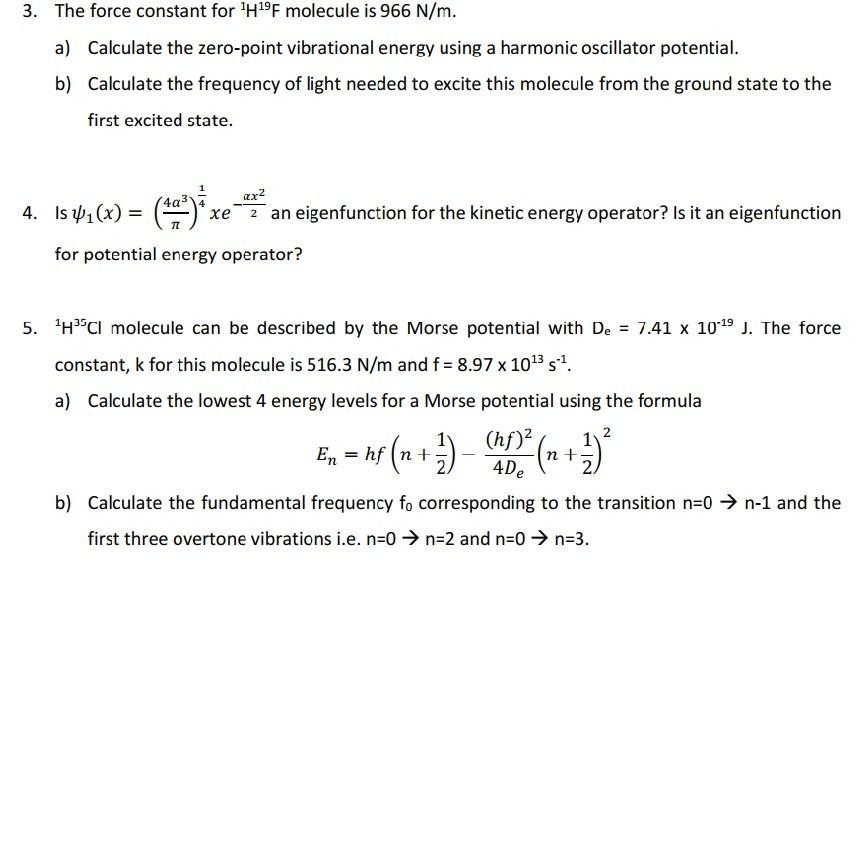4&5 only thnkyouu :) 3. The force constant for 119F molecule is 966 N/m. a) Calculate the zero-point vibrational energy using a harmonic oscillator potential. b) Calculate the frequency of light needed to excite this molecule from the ground state to the first excited state. 4. Is 41(x) = *xe 2 an eigenfunction for the kinetic energy operator? Is it an eigenfunction for potential energy operator? 5. HCI molecule can be described by the Morse potential with De = 7.41...